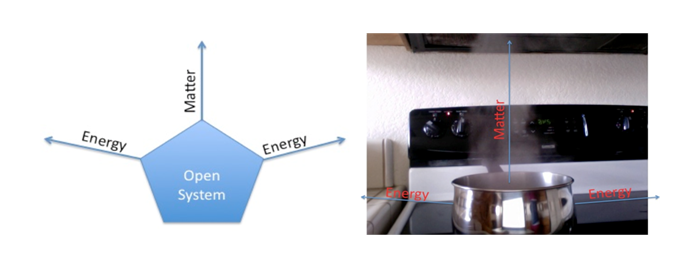# First Law of Thermodynamics with open and closed system Examples

## First Law

Energy cannot be created nor destroyed in any isolated thermodynamics system but it can be changed from one form like heat into another form like work or internal energy or both. First law of thermodynamics follow the law of conservation of energy and it state that energy cannot be created nor be destroyed but it can be converted from one form to another form. From first law of thermodynamics it can be said that the internal energy of the system remains same and it is equal to the heat provided to the system minus the work done by the system.

## Open System

Open system are those in which mass and heat can cross the boundaries of the system. Example of first law of thermodynamics for open system can be seen in pumps, turbines and heat exchanges where heat and mass cross the boundaries of the system. In such application the internal energy of the system is equal to the heat provided to the system minus the work by the system. In these system mass in slowing out of system boundaries which means a lot of energy or heat is getting wasted with mass but the system is in equilibrium as the required amount of heat is also being provided with inlet mass.

In the following example water is billing in a boiler with the top of the boiler wide open. Heat is providing to the system with the help of an external source (fire) and as a result of it internal energy of the water is increasing. With the increase in internal energy of water a lot of heat is wasted from the boilers walls and from the vapours going out of the boiler. This water boiling system is open system as heat and mass both are going out of the system## Closed System

Closed system is one in which mass of the system cannot leave the system boundaries but the heat can. First of thermodynamics has some example for closed system in which head enters and exist the system and system also give some work output but the system mass does not leave its boundaries one of this example is piston cylinder arrangement where heat provided is converted into motion of piston.

In the below example when boiler top is covered and steam is not allowed to leave the system the only heat can leave the system through the boundaries of system means from the walls of the boilers